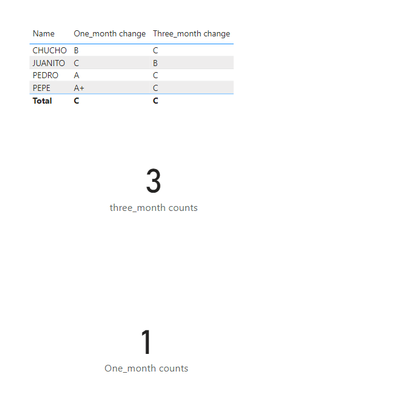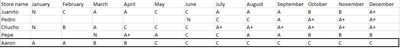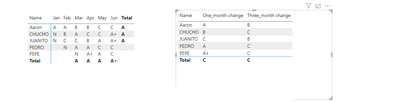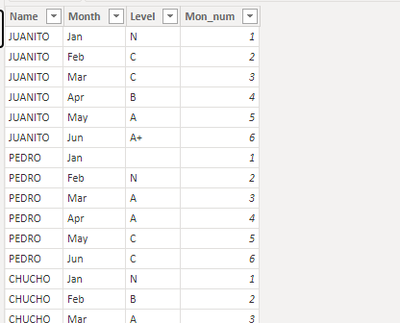cancel
Showing results for
Did you mean:Frequent Visitor

## Count class changes and abandon rate

Hi guys I really don't know how to name this question but here is what im trying to do.

I have stores labeled by a class A+, A, B, C and N every time we open a new store we name them N every month it changes from N to any other class based on their results, first I want to know if I can count this jumps from N-C or N-B,etc. In the first month, after three months of this change I need to see if they still at a good class. and point the ones that are "abandoned" the ones that are C class and the ones that are good A, A+.

Example:Total of N stores that changed to C in their first month of operation =1 juanito's

Total of N stores abandoned "C- class" in their third month of operation = 3, Pedro's Chucho's and Pepe´s stores

Thanks a lot for the help! I really appreciate it.

1 ACCEPTED SOLUTIONCommunity Support

Please refer to the following measures.

``````One_month change = var a=CALCULATE(MAX('Table'[Mon_num]),'Table'[Level]="N")
var b=IF(a<>BLANK(),CALCULATE(MAX([Level]),'Table'[Mon_num]=a+1),CALCULATE(MAX([Level]),'Table'[Mon_num]=MIN('Table'[Mon_num])))
return IF("N" in VALUES('Table'[Level])&&b="C",1,0)

Three_month change = var a=CALCULATE(MAX('Table'[Mon_num]),'Table'[Level]="N")
var b=IF(a<>BLANK(),CALCULATE(MAX([Level]),'Table'[Mon_num]=a+3),CALCULATE(MAX([Level]),'Table'[Mon_num]=MIN('Table'[Mon_num])+2))
return IF("N" in VALUES('Table'[Level])&&b="C",1,0)``````
``````One_month counts = SUMX(VALUES('Table'[Name]),[One_month change])

three_month counts = SUMX(VALUES('Table'[Name]),[Three_month change])``````

OutputBest Regards!

Yolo Zhu

If this post helps, then please consider Accept it as the solution to help the other members find it more quickly.

6 REPLIES 6Super User

HI @RicardoNeri
Not sure if can be helpful to youCommunity Support

Hi @RicardoNeri

You can refer to the following measures

1.Create two measures to calculate the fist month and the third month level

``````One_month change = var a=CALCULATE(MAX('Table'[Mon_num]),'Table'[Level]="N")
var b=CALCULATE(MAX([Level]),'Table'[Mon_num]=a+1)
return b

Three_month change = var a=CALCULATE(MAX('Table'[Mon_num]),'Table'[Level]="N")
var b=CALCULATE(MAX([Level]),'Table'[Mon_num]=a+3)
return b``````

2.Then create two other measures to count the fist month counts and the third month counts

``````One_month counts = COUNTROWS( FILTER(VALUES('Table'[Name]),[One_month change]="C"))

three_month counts = COUNTROWS( FILTER(VALUES('Table'[Name]),[Three_month change]="C"))``````

Then display the count measuresBest Regards!

Yolo Zhu

If this post helps, then please consider Accept it as the solution to help the other members find it more quickly.Frequent Visitor

Hello Yolo Zhu I tried to use your commands and they work but I think I just messed up a bit and here is why I thinks so. Not all workers starts from N class because they have been working longer from the data I have so they can start with other class, here is an example.Here Aaron appears as he had his first month store as C but the thing is that he was never in the N categorySo in the mayority of cases I have data like Aaron's. What can I do?

Thanks a lot @v-xinruzhu-msft  for the helpCommunity Support

I don't quite understand how to define the first month of store opening, at first I understood that the first month after a certain store is marked N is the month in which the store is open, but you said this Aaron store, then his first month should be January?

Refer to the above understanding, you can refer to the following two measures.

``````One_month change = var a=CALCULATE(MAX('Table'[Mon_num]),'Table'[Level]="N")
var b=IF(a<>BLANK(),CALCULATE(MAX([Level]),'Table'[Mon_num]=a+1),CALCULATE(MAX([Level]),'Table'[Mon_num]=MIN('Table'[Mon_num])))
return b

Three_month change = var a=CALCULATE(MAX('Table'[Mon_num]),'Table'[Level]="N")
var b=IF(a<>BLANK(),CALCULATE(MAX([Level]),'Table'[Mon_num]=a+3),CALCULATE(MAX([Level]),'Table'[Mon_num]=MIN('Table'[Mon_num])+2))
return b``````I  add a number field [Month_num  in the sample data you providedBest Regards!

Yolo ZhuFrequent Visitor

The thing with Aaron store lets say the data sheet I showed is from the year 2022 but Aaron started working with us in 2021 thats why he starts the year with a classification different from N, in 2022 with the data sheet we know. Chucho started in february thats why he has the N class in february and nothing in january, what I want to do Its like you did count the steps like chucho and the others but ignoring cases like Aaron that never started as N in the year so my table only shows this cases and those jumps.

Here is the example lets say Aaron started january as C class instead of ANow the formula takes january as the first month and adds it to chucho and the others which is wrong, I dont want it to take this case in consideration because Aaron was never in the N class I just want chucho and the others that joined this year working with us. If they never were in the N class in the year just ignore them "Aaron" and just take into consideration the ones that were N in the year "Chucho" "Juanito"

Thanks a lot for your time Yolo Zhu I really appreciated it!Community Support

Please refer to the following measures.

``````One_month change = var a=CALCULATE(MAX('Table'[Mon_num]),'Table'[Level]="N")
var b=IF(a<>BLANK(),CALCULATE(MAX([Level]),'Table'[Mon_num]=a+1),CALCULATE(MAX([Level]),'Table'[Mon_num]=MIN('Table'[Mon_num])))
return IF("N" in VALUES('Table'[Level])&&b="C",1,0)

Three_month change = var a=CALCULATE(MAX('Table'[Mon_num]),'Table'[Level]="N")
var b=IF(a<>BLANK(),CALCULATE(MAX([Level]),'Table'[Mon_num]=a+3),CALCULATE(MAX([Level]),'Table'[Mon_num]=MIN('Table'[Mon_num])+2))
return IF("N" in VALUES('Table'[Level])&&b="C",1,0)``````
``````One_month counts = SUMX(VALUES('Table'[Name]),[One_month change])

three_month counts = SUMX(VALUES('Table'[Name]),[Three_month change])``````

OutputBest Regards!

Yolo Zhu

If this post helps, then please consider Accept it as the solution to help the other members find it more quickly.